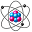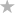#### File Exchange > Data Analysis >    Radiometric Geochronology AnalysisAuthor:
OriginLab Technical Support
3/9/2022
Last Update:
6/8/2022
170
Total Ratings:
0
File Size:
556 KB
Average Rating:File Name:
File Version:
1.13
Minimum Versions:
Summary:

Description:

Purpose

This App can be used to analyze radiometric geochronological data (Pb-Pb, Ar-Ar, Rb-Sr, Sm-Nd, Lu-Hf, Re-Os, U-Th-He, U-Pb). It includes several tools, including isochron, weighted mean, kernel density estimate, cumulative age distribution, york fit and concordia diagram.

Installation

The app requires R software (minimum required version 3.4.1) and package (IsoplotR). If R is not yet installed on your computer, please follow these steps.

1. Run Origin as administrator. Download the opx file and install it by choosing Tools->Package Manager->Tools->Install a Package.
3. For the first time you launch the App, it downloads dependent R packages. Wait a few minutes until it is completed.

If you reinstall or upgrade R after installing the app, it would not work properly. Please reinstall the app.

Operation

• Input Data

1. Choose Isotopic Data type (Pb-Pb, Ar-Ar, Rb-Sr, Sm-Nd, Lu-Hf, Re-Os, U-Th-He).
2. Choose Input Format from Normal, Inverse(X, s[X], Y, s[Y], &rho[X,Y]) and Three ratios(X, s[X], Y, s[Y], Z, s[Z]).
3. Specify input data range.
4. Select Input Errors type from 1 se (abs), 2 se (abs), 1 se (%) and 2 se(%).

Notes: X, Y and Z are the isotopic (ratio) measurements. s[X], s[Y], s[Z] are analytical uncertainties (standard errors). &rho[X,Y] is the error correlation.

• Dialog Options

Isochron

• Model
• Error-weighted least squares regression:This algorithm takes into account the analytical uncertainties and error correlations, under the assumption that the scatter between the data points is solely caused by the analytical uncertainty.
• Ordinary least squares regression: This algorithm  ignores the analytical uncertainties.
• Error-weighted least squares with overdispersion term: Instead of attributing any overdispersion to underestimated analytical uncertainties, one can also attribute it to the presence of geological uncertainty, which manifests itself as an added (co)variance term.
• Inverse isochron: toggles between normal and inverse isochrons.

Weighted Mean

• Alpha: The confidence limits of the error bars.
• Common Pb Correction:
• None: No correction.
• Nominal: Use the stored Pb-composition.
• Isochron: Remove the common Pb by projecting the data along an inverse isochron. Note: choosing this option introduces a degree of circularity in the weighted age calculation. In this case the weighted mean plot just serves as a way to visualise the residuals of the data around the isochron, and one should be careful not to over-interpret the numerical output.
• Isochron to Intercept: Calculate the initial 40Ar/36Ar, 40Ca/44Ca, 207Pb/204Pb, 87Sr/86Sr, 143Nd/144Nd, 187Os/188Os, 230Th/232Th, 176Hf/177Hf or 204Pb/208Pb ratio from an isochron fit. If this option is uncheck,  use the default values.
• Reject Outliers: Indicate whether outliers should be detected and rejected using Chauvenet&rsquos Criterion.
• Random Effects: Compute the weighted mean using a random effects model with two parameters: the mean and the dispersion.

Kernel Density Estimate

• Adaptive KDE: If checked, the adaptive KDE modifier of Abramson is used.
• Common Pb Correction/Isochron to Intercept: Follow the same instructions as Weighted Mean

Cumulative Age Distribution

• Common Pb Correction/Isochron to Intercept: Follow the same instructions as Weighted Mean

York Fit

• Alpha: Cutoff value for confidence intervals.

Concordia

• Age:
• Calculate no age: Plot the data without calculating an age.
• Calculate concordia age: Fit a concordia composition and age.
• Calcuate discordia (model-1) age: Fit a discordia line through the data using the maximum likelihood algorithm of Ludwig, which assumes that the scatter of the data is solely due to the analytical uncertainties.
• Calcuate discordia (model-2) age: Fit a discordia line ignoring the analytical uncertainties.
• Calcuate discordia (model-3) age: Fit a discordia line using a modified maximum likelihood algorithm that includes accounts for any overdispersion by adding a geological (co)variance term.
• Concordia Type:
• Wetherill: 206Pb/238U vs. 207Pb/235U
• Tera-Wasserburg: 207Pb/206Pb vs. 238U/206Pb
• U-Th-Pb concordia: 208Pb/232Th vs. 206Pb/238U (only available if input format is 7th or 8th type)
• Alpha:Probability cutoff for the error ellipses and confidence intervals.

Sample OPJU File

This app provides a sample OPJU file.  Right click the App icon in the Apps Gallery window, and choose Show Samples Folder from the short-cut menu. A folder will open. Drag-and-drop the project file Radiometric Geochronology Analysis Sample.opju from the folder onto Origin. The Notes window in the project shows detailed steps.
Note: If you wish to save the OPJU after changing, it is recommended that you save to a different folder location (e.g. User Files Folder).

Reference

https://cran.r-project.org/web/packages/IsoplotR/index.html